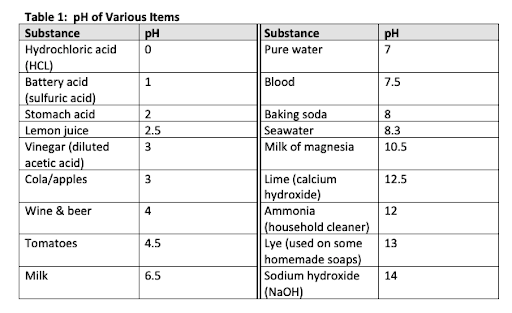# Question 25-Science Practice Test for the ACT

In Figure 1 in the attached text, what volume of base was needed to reach the equivalence point?

Many of the household products we use, as well as various foods, are either acidic or basic, however, we can only consume those foods which are weakly acidic or basic. If we ingest strong acids or bases, such as some chemical cleaning solutions, we could compromise our health and even die.

Water is made up of acids (H+, hydrogen ions) and bases (OHB, hydroxide ions), and its formula is H2O. In pure water, the number of H+ ions is equal to the number of OHB ions. When a substance has more H+ ions it is an acid and conversely, when a substance has more OHB ions it is base. We use pH (the power of hydrogen) to measure the concentration of a substance—to know how acidic or basic the substance is. Put another way, pH measures the amount of H+ and OHB ions.

We use the pH scale, which ranges from 0B14 to classify acids and bases. Pure water is neutral with a pH of 7. pH values below 7 are acidic (0 being the strongest acidity) and those above 7 are basic (14 being the strongest basicity). pH is measured with either indicator paper, which is treated with chemical indicators that vary in colour according to pH, or a pH meter, which is an electronic device with an attached probe. The probe contains an acidic solution covered by glass that measures the migration of H+ ions across it. The probe is inserted into an unknown solution and, if this solution has a different pH than the solution in the probe, an electric potential results, which is registered on the meter.

Andrea and Ryan are partners in their chemistry lab and their TA had assigned them a project on acids and bases last week. For their first experiment, they determined the pH of various items using a pH meter. For their second experiment, they determined the equivalence points of mixed acidic and basic solutions. They added either a strong base or acid, drip by drip, to another strong acid or base, respectively. After each drip was added, they measured the pH of the solution and recorded the value.

Titration curves were graphed for each dyad of solutions to determine the equivalence point (see Figure 1 which is an example of one of their titration curves). The equivalence point is when the two solutions have been mixed in exactly equal proportions of acid and base. Titration curves not only show you the equivalence point but also what volume of solution is needed to turn an originally acidic or basic solution into a basic or acid solution, respectively. For example, as a strong base is slowly added to a strong acid, the acidic solution gradually becomes a weak acid and then a weak base, and finally a strong base (Figure 1).Create a Profile